Maths-
General
Easy

Question

#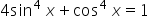if

##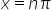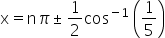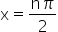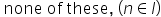Hint:

## The correct answer is:### Here, we have to find the the general solution for xFirstly, we have given equation,So we can write,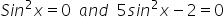x = n π and sin2x =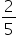-2 sin2 x =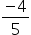1 – 2 sin2 x =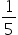Cos 2x =2x = 2n π ± cos-1 ()X = n π ±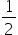cos-1 () Therefore, the correct answer is x = n π and x = n π ±cos-1 (). Both options will correct.

In this question, we have to find the general solution of x. Here more than one option will correct. Remember the rules for finding the general solution.#### With Turito Foundation.#### Get an Expert Advice From Turito.